﻿ Kartazion

#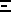Kartazion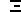## Scientific Research & Quantum Engineering - Cryptoassets

Native Kartazion \$KZN cryptocurrency on Solana

Wrapped Kartazion \$WKZN cryptocurrency on Binance_____________

## Welcome to Universal Theory 1.0

Explain Quantum Physics With a Single-Particle in Motion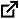|

|

|

|

_____________
______
___

## Welcome to Universal Theory 2.0

Once Upon a Time the Universe:
Anharmonic Oscillator________________

________________

I - Foreword

Fundamental reminder Volume I:

Fundamental reminder Volume I "Explain Quantum Physics With aSingle-Particle in Motion" ISBN 9780244217730

Based on the optical illusion, oscillating or alternating at the speed of light a 5mm particle from position A to position B spaced 10cm apart, would beseen as two visible points as a fixed and static appearance. The most important thing is to be able to mark a certain stop on each position of A and B, and to travel between them almost instantaneously. This technique describes an oscillator and is the foundation of all the theory developed in this publication.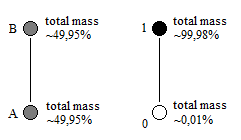In conclusion and in volume I, the particle only oscillates between its point of origin 0 and the matrix. The matrix being the area where the multiple positions of the particle are to be represented by scanning or iteration of itself. This anharmonic oscillation of one and the same particle at very high frequency establishes the mechanical link between the functioning of the atom and the universe.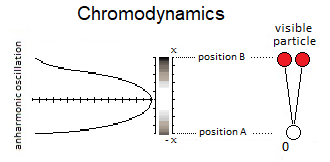Moreover, this oscillation of the particle itself creates the quantum suerposition states, as well as the Qbits which use this principle of particle duplication. Example and animation (follow QR-code / anharmonic-oscillator / readme): https://github.com/KartazionIntroduction:

The functioning of our universe and atomic is based on the oscillation of the particle itself. This mechanism is a classical an.harmonic oscillator and usesa linear oscillation of the particle, where the energy can be represented by the graph of a potential well. In a more exhaustive development than Volume I, this anharmonic oscillation therefore occurs between matter and antimatter, followed by gravitational singularity avoidance (the previous model of Volume I being an oscillation between its point of origin and the matrix. In volume II the important kinetics of the particle leads to an singularity avoidance). This singularity avoidance is of the supermassive black hole and / or big-bang type. On the other hand, and at total rest at x=0, this particle is at the bottom of the potential well, at the lowest level, at the singularity, namely the total collapse of the universe which represents a state of true vacuum. But again and by a more sustained oscillation a singularity avoidance which is opposite of rest creates the levitation of the particle into higher energy levels in its potential well. This singularity avoidance allows access to the current false vacuum without falling at the bottom of the well, thanks to the Kinetic Energy or the inertia of the particle. The role of the singularity in our model is fundamental and implies that it is the source and driver of the actual known result of quantum and cosmological fluctuations in relation to the motion of the particle.

Harmonic Oscillator vs Anharmonic Oscillator. Indeed the difference is made here and is between the ideal harmonic layout and the absolut anharmonic layout of the energy potential well. In the case of singularity avoidance due to the high Kinetic Energy of the particle, the plot of the curve of the potential well tends towards a harmonic shape rather than an anharmonic one. Moreover the anharmonic implication can be double: the variation of speed of the particle followed by the shape of the potential well due to the singularity can involve this anharmonicity plus morse. In the following illustration, the integration of the singularity at x=0 is not yet represented.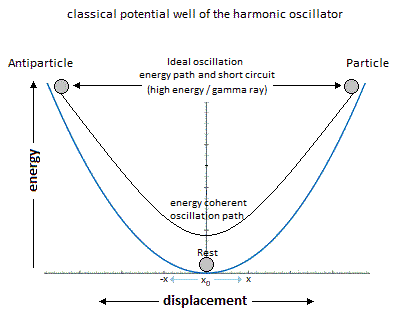If the energy potential well has a curved shape, it represents the shape of the quantity of energy delivered to the particle along x. Which does not mean that the particle moves in a half-moon. The particle moves nice and well ina straight line in a radiative or linear way. In the previous graph we can distinguish three types of possible interpretations of the states and paths of the particle. At 1 and at the bottom of the harmonic energy potential well at x=0, we have the rest of the motionless particle (or ZPE). In 2 and with Kinetic Energy applied to the particle is at x=0 the maximum speed of the particle and allows singularity avoidance by drawing a harmonic curve. In 3 it should be noted that the ideal trajectory of the particle is of the short circuit type between matter and antimatter and would trace a straight line, i.e. the shortest path in the potential well. This utopian energy path is actually impossible for the particle to take. However, the annihilation of the electron / positron pair into a gammaphoton should indicate a short-circuit type trajectory, and ultimately definethe characteristic of a boson. In the following illustration an integration of the gravitational singularity disturbs the harmonic shape of the potential well. The greater the kinetics of the particle in its oscillation, the more the shape of the potential well tends towards harmony by avoidance of gravitational singularity. But if the kinetics of the particle is weak, then the particle falls at the bottom of this gravitational singularity and traces the anharmonicity of the potential well.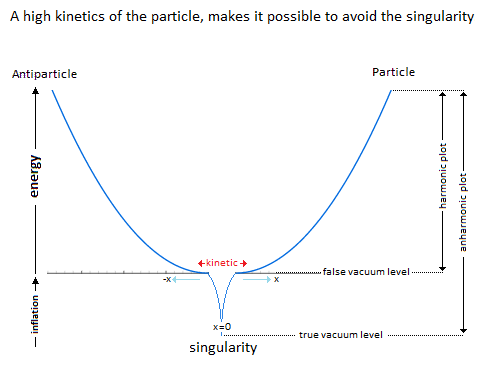In a second part of this introduction, it is a question of the singularity avoidance where the particle through its path, traces the Higgs field. The Potential Energy is in relation to the depth of the singularity, because to pass over a black hole would have for value an enormous Potential Energy, and would trace the curve of the potential of Higgs of it.II - Gravitational oscillator

Perpetual Oscillation & Ideal Distribution of Energies

Perpetual motion is utopian. But in our case it represents an oscillation without mechanical constraint followed by the principle of energy conservation. In an interpretation of classical mechanics, the an.harmonic oscillation of the particle can be represented through a gravitational oscillator. It is initially used gravity to subject by attraction a movement to the particle. Here is the example chosen of an oscillation of the particle which uses the same direction as the vector of gravity to be able to move. Its description is based on the "hole through the earth" which does not really have an example in French but which is nevertheless well studied forits principle. Here is his summary explanation: "If you drilled a hole in theaxis of the Earth from pole to pole, and inserted a long, thin vacuumchamber into it, and then dropped an object into one end of that chamber, it would fall into the hole, picking up speed and it would move very fast whenit reached the center of the Earth so it would continue until it reached the other pole where it would stop, then fall back "bounce" to come back andstart again perpetually." (google 'hole through the earth simple harmonic motion').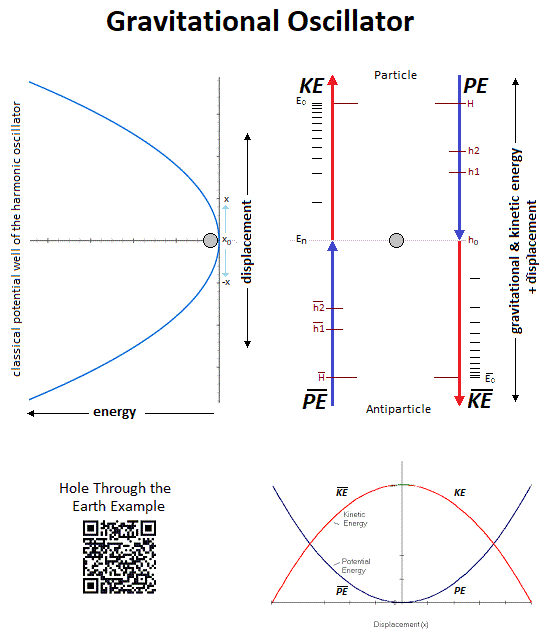This linear gravitational oscillator uses a particle of mass m oscillating vertically along the gravity vector G. The oscillation has two phases. The first is the falling phase of the particle with its Potential Energy PE and thesecond is the reverse phase which corresponds to the Kinetic Energy KE. Example: It is from the center of the Earth that which is in Potential Energyis transformed into Kinetic Energy and is reversed at the level of the point 0 or x=0 and vice-versa.

Kinetic Energy & Potential Energy

In conclusion, for the gravitational oscillator presented above, the distribution of the two energies follows the following diagram and operates according to the linear displacement of the particle, i.e. the alternation of the Potential Energy PE with the Kinetic Energy KE.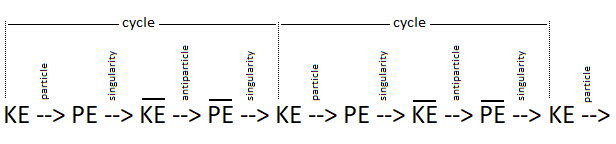Potential Energy - Kinetic Energy = 0

Source of quantum fluctuations & gravitational singularity

In our model the source and the material existence as we know it is based on the implication of the singularity of the big-bang type for the universeand of the supermassive black hole type for the functioning of the galaxies (and other theoretical singularity as for that of the center of an atom). In other words, the divergent evolution of the volume of the singularity is responsible for the matter that remains in its space-time. As gravity (Potential Energy) pulls the particle towards the core of the singularity, andwho approaches it to fall inside, is then suddenly expelled by pure energy emanating from that same singularity. Indeed the singularity generates in 1 the gravitational attraction noted Potential Energy PE and in 2 the prediction that the source of energy is by scratching and / or astrophysical relativistic jet noted Kinetic Energy KE emanates from this same singularity. Moreover in 3 it should be noted that the singularity generates eletric charges on the particle.

In a more speculative and exotic definition and given our knowledge of the singularity in terms of gravitation followed by its time dilation, we could associate the center of the earth as such. Indeed for the particle which oscillates in this direction it becomes easy to imagine an singularity avoidance at the level of the Earth's core in the same way as the supermassive black hole in relation to its galaxy.

III - Singularity avoidance

Singularity avoidance is due to the high Kinetic Energy of the particle. At x=0 when the particle is going as fast as possible, its Kinetic Energy allows it not to fall at the bottom of the well at the level of the gravitational singularity. Gravity (Potential Energy) corresponds to the matter attracted towards this singularity, while energy pushes it back. In our case it is simply a particle rather than a cluster of matter.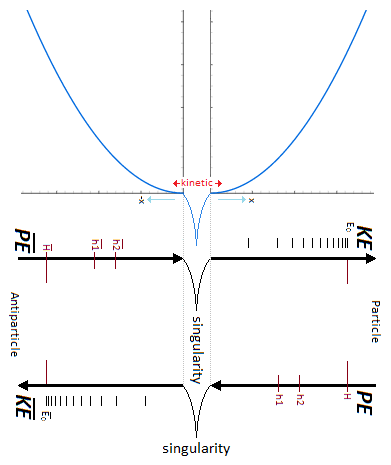Higgs field

Significant particle kinetics avoids the singularity, and the path the particle takes above this singularity determines the plot of the Higgs fieldIn a second time the theoretical junction between the singularity avoidance and the Higgs field can be done by the enormous Potential Energy. Indeed, the Potential Energy being that in relation to the depth of the gravitational singularity gives the particle a much greater mass, or even a maximum value.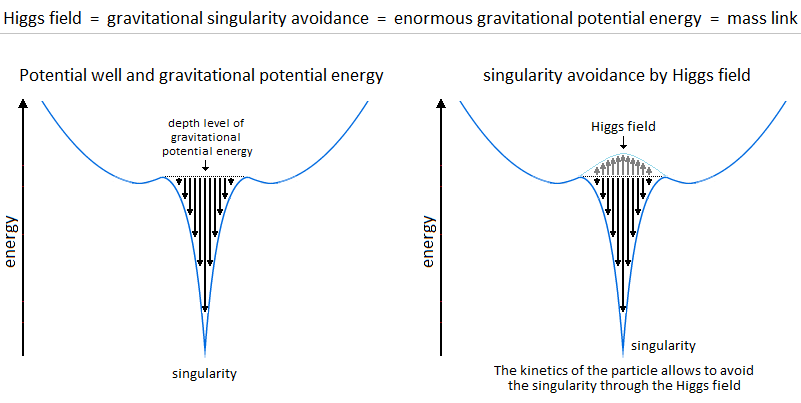The Higgs field and potential are also well used to be able to represent the metastability of the universe, as well as its interpretation as a quantum particle for its origin of the mass.

Singularity avoidance = enormous Potential Energy = Higgs mechanism = mass link

Second interpretation of singularity avoidance:

It should be understood that in the case of slowed kinetics, the particle will then tend to pass through the virtual slit of the tunnel effect instead of the singularity avoidance by the Higgs field and finds itself at the level of the black hole horizon event. This being said, this does not mean that the particle will finally reach true vacuum because kinetic energy is then sent or subjected to the particle in order to be able to eject it again into the upper harmonic well of our universe. This is to be considered as a singularity avoidance at the horizon event of a black hole.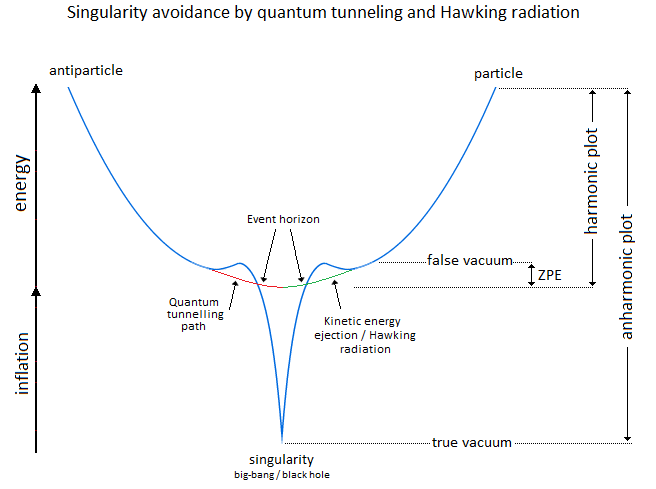Orbit, inertia, ZPE & potential barrier

We indicate a Potential Energy barrier around gravitational singularities. This barrier is due to the deformation of the space-time curvature. At least a difference in level where the Potential Energy is felt around the mass object. Without Kinetic Energy, the inertia of the object (or particle) allows it to slide along the potential barrier that defines the path of the orbit around an even more massive object. In other words, the deformation of the space-time curvature due to an average object is felt at the level of the heights of the energies, and creates a barrier.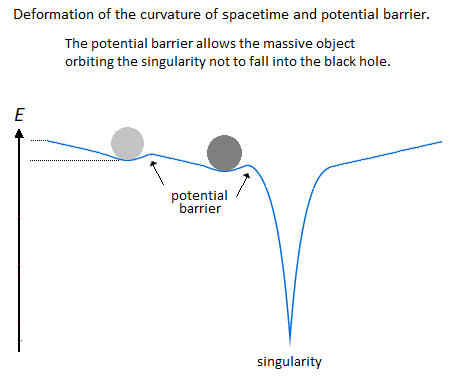The ZPE (Zero Point Energy) represents the initial perturbation of the particle. At the quantum level this means that when the particle is at rest at the bottom of the harmonic potential well, the particle undergoes an oscillating disturbance. In cosmology this disturbance represents the inertial movement as an object orbiting around the gravitational singularity / massive object. In other words, the initial quantum disturbance of the Zero Point Energy (ZPE) corresponds to the cosmological movement of the object / particle located in the false vacuum in orbit around the gravitational singularity. Deformation also occurs for massive like stars objects.

Vacuum metastability

Vacuum metastability is determined by the amount of Kinetic Energy applied to the particle to trace its potential field. If the Kinetic Energy of the particle is sufficient and therefore if the range of the energy condition allows the energy potential barrier to be passed, then singularity avoidance occurs; But during an attenuation of the kinetics of the particle or even a total stop of the inertia, that will cause by its amount of lower energy its fall towards the singularity and will reach the true vacuum. In other words, the inertial disturbance of the ZPE of the particle makes it possible to remain in the false vacuum by inertia, until a fictitious drop in this energy slows it down and then causes it to fall through a virtual slit to reach the true vacuum.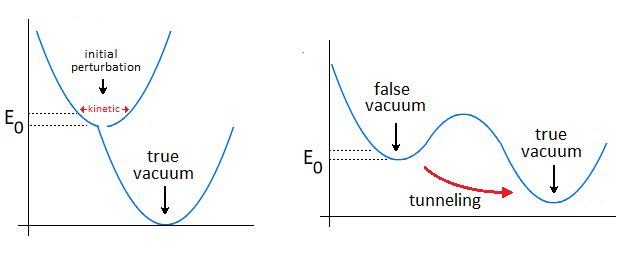Graviton & Potential Energy

According to the units of Planck, the number and the quantity of gravitons put end to end (point to point) along the vector of gravity G, then gives the total of the energy of gravitational potential. Each increment / decrement of the graviton, is obtained by adding or subtracting a linear total of energy quanta, which can be represented by the size of the particle. It becomes easy to take into account the number of gravitons accumulated over the length of a ray noted in nm where this ray is parallel to the length of the vector G inorder to be able to have a relationship between the number of gravitons in relation to the Potential Energy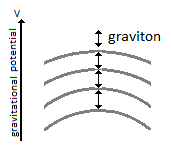IV - Asymmetry CP matter antimatter

Most important is the role and the why of antimatter. As we can see the gravitational oscillator looks like a balanced perpetual motion without mechanical constraints. It is precisely on this side of the balance without mechanical constraint of the oscillation, that it makes that between Kinetic Energy and gravitation (Potential Energy) the role of the antimatter becomes important. This allows the particle to simply bounce (due to deceleration from depletion of the particle's Kinetic Energy) to turn around using Potential Energy. There is therefore no impact of the particle that occurs in its cycle of oscillation. The Dirac Sea is a perfect representation of what the electron becomes in the depths of energy. Dirac predicts antimatter and the positron. We must therefore imply an anti-inflation followed by its anti-universe. This therefore explains why we do not find the expected antimatter in the matter side of the universe, because antimatter is indeed found on the anti-universe side. In conclusion during a high energy collision, the annihilation of the pairs of particles make detect thanks to the gamma photon the presence of antimatter through the space-time.

Symmetry breaking CP & arrow of time T

We understand that the symmetry breaking is located at x=0 at the level of the gravitational singularity. There is therefore a link between the Higgs potential and the symmetry breaking around x=0. If the charge and the parity CP are inter-changed following a linear movement of the particle either from bottom to top, then the arrow of time is perpendicular and flows for example from left to right.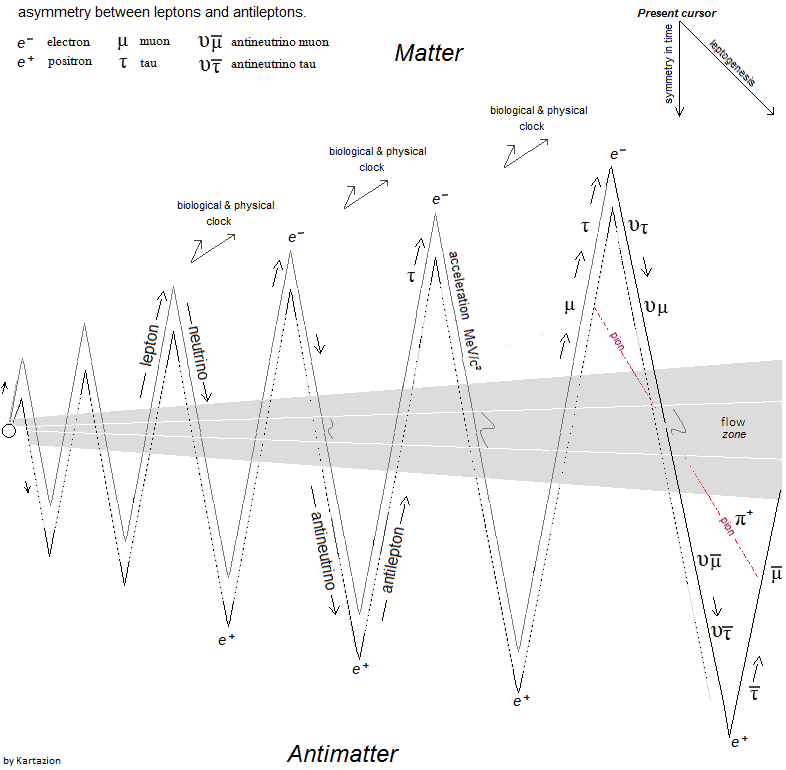Path of the particle, quantum fluctuation & Dark matter

Based on the functioning of the mechanism of the anharmonic oscillator, involves during the movement of the particle, to make it go through different physical stages due to its high speed of displacement between matter and antimatter. We can also talk about the particle's energy flow to express the different cosmological or quantum states observed (Kasimir effect, etc.). The path of the particle is therefore linear and forms round trips. The path of the particle is therefore radiative. When the particle reaches a sufficient speed, a transformation into mass energy by the equivalence principle occurs. A small trace of this energy is found in vacuum and represents quantum vacuum energy. The successive addition of vacuum energy gives dark matter. In other words, the convergence of the energy flow (quantum fluctuation) of the quantum vacuum, in a more restricted space, close to the singularity, then in turn becomes dark matter. At its opposite and the opposite of the singularity, there is matter expressed by quantum chromodynamics. Dark matter and quantum vacuum energy / quantum fluctuation is produced with the condition of the particle moving at very high speeds. Here is an example of the absolute path taken by the particle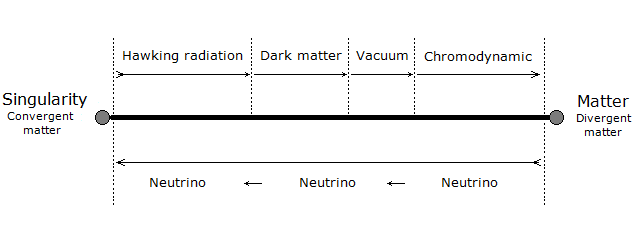Here is a simple energy potential well to explain dark matter and quantum vacuum and quantum chromodynamics in relation to the oscillator energy potential well: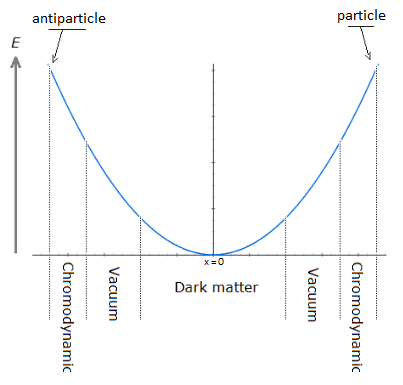Dark energy

Dark energy corresponds to the increase in the size of the potential well. In the gravitational oscillator, the incrementation of the particle, that is to say a height of radiation greater than the previous height in the potential well, is produced by additional energy called dark energy; And which makes the particle advance further and further from its gravitational source. The example of a galaxy, where inside does not know an expansion of size inrelation to its super massive black hole, uses a constant amount of Kinetic Energy in relation to gravity (Potential Energy). The increase in this Kinetic Energy that we have seen so far, then becomes dark energy, while it is onlya amount of energy greater than the previous energy impulse. In other words, to move the particle further and further away from the singularity (i.e. big-bang) with the gravitational oscillator, more energy is needed. This extra amount of energy is dark energy.

V - Atomic structure

Quantum superposition

Schrödinger's Cat is a thought experiment that illustrates the result of the quantum superposition paradox. If the particle is on A, then it cannot be on B. But at very high frequencies the particle has almost a 50% chance of being on A and B at the same time, hence the superposition state. If you were to randomly choose a position between A and B, then you would either have the particle in the hand (alive) or no particle in the hand, hence the cat experiment. In Prediction; The superposition of quantum states is at least straddling matter and antimatter before being detected on the matter side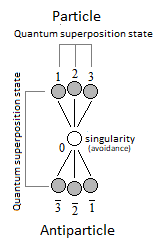Quantum ChromoDynamics

Quantum ChromoDynamics (QCD) would only be a duplication of the particle itself. It describes the mechanics of the quark superposition transition. In other words, quantum chromodynamics is the alternation of the particle itself to form the different combinations of quarks.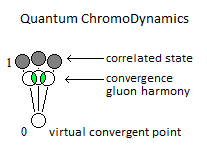The implication of radiation is simply due to the fact that the particle in its oscillation is linear. The direction of the work of the particle is along thevector of gravity, namely parallel to it. The gravitational oscillator has two types of radiation. First there is the vertical radiation, called fermionic, i.e. the normal oscillation of the particle from bottom to top and from top to bottom; And there is the horizontal radiation, or bosonic radiation which is not the oscillator. For the fermionic radiation and with the example of the lepton we have a movement of the particle from bottom to top in the oscillator which makes it possible to transport an electric charge to thesurface of the sea of energy. In the opposite direction, either from top to bottom the particle goes down again in neutrino, or with a neutral charge. IOW this model, there is the link between Kinetic Energy and electric charge, and the neutrino follows the Potential Energy, (gravity). Bosonic radiation, in its analogous interpretation, emits a kind of electric arc thatoccurs horizontally.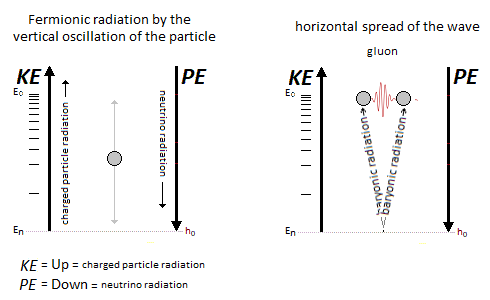Atom & quantum atom

The quantum atom is basically composed of quantum leap of the particle between matter and anti-matter. These jumps correspond to the Bottom-up oscillation and have an almost instantaneous value. They can for example be of the order of a few million or a few billion jumps in a nanosecond. The Pauli exclusion is always respected because there is only one particle present per atom created by a reiteration of its position in different and unique states.

Pure quantum atom. The pure quantum atom is non-isotropic. It corresponds to the two choices which is that of the neutron N or the proton P. The reiteration in a series of Neutrons N followed by Protons P (same number of N as of P) is a pure atom. The atomic model described here represents the synopsis of the logical sequence of the oscillation mechanism of a single moving particle. Here is the diagram of the anharmonic characteristic of the particle. Its oscillation is located between matter and antimatter, where between two its acceleration would then be almost instantaneous by singularity avoidance. We can see by the anharmonic oscillator the classic version of the internuclear distance followed by the morse potentials to be able to give the energy wanted to the particle.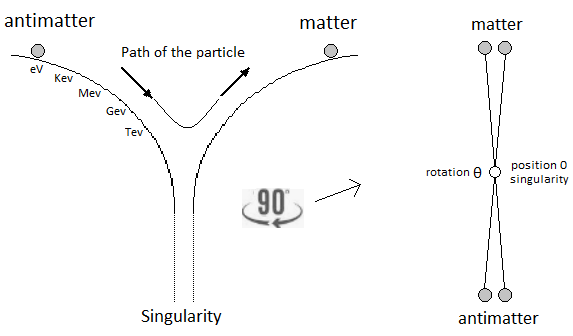The neutrino represents the particle without electric charge and is electrically neutral. The neutrino has a direct relationship with that of Potential Energy. On the contrary, when the particle is emitted by Kinetic Energy, the latter carries an electric charge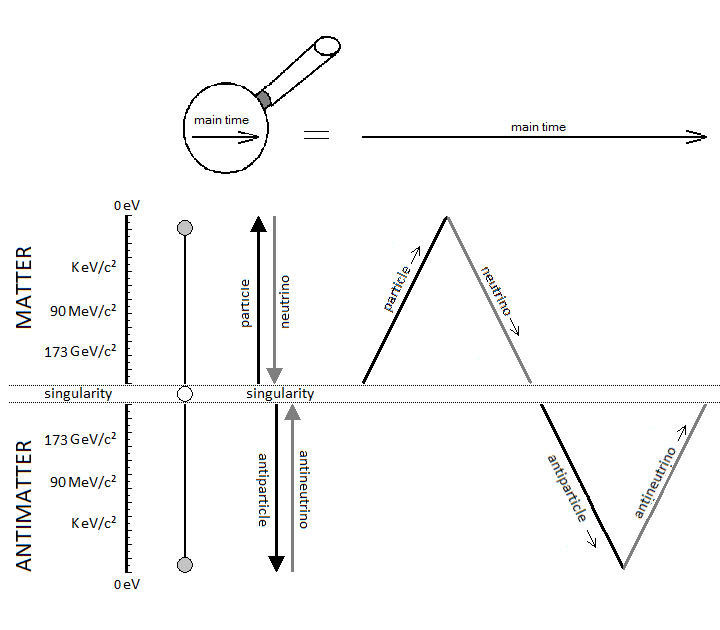The dosage of the Kinetic Energy makes it possible to deliver the amount of energy necessary in term of electron-volt.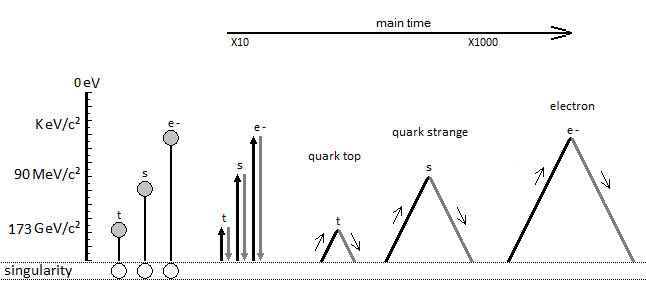Thanks to the principle of reiteration, and if you had the choice between neutron and proton, the probability of finding an N neutron followed by a Pproton like NP or NPN in the atomic nuclei is substantial. Which brings us, and in relation to the atomic signature, to the conclusion of a composition rich in Deuterium, Tritium and Helium 4-5-6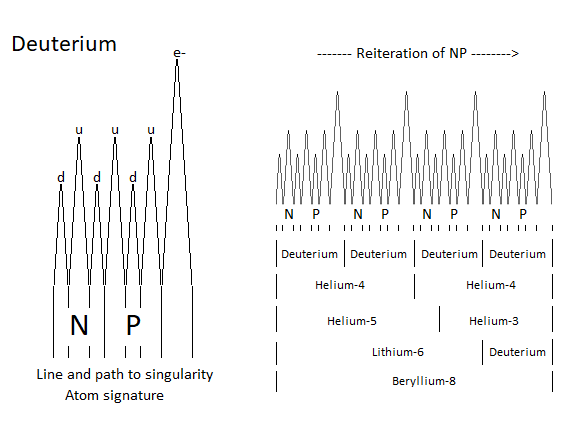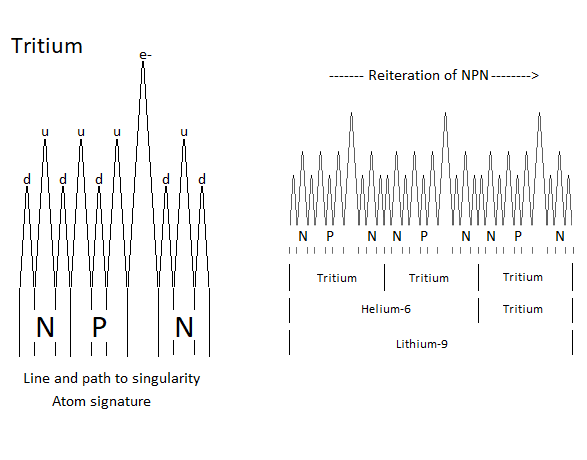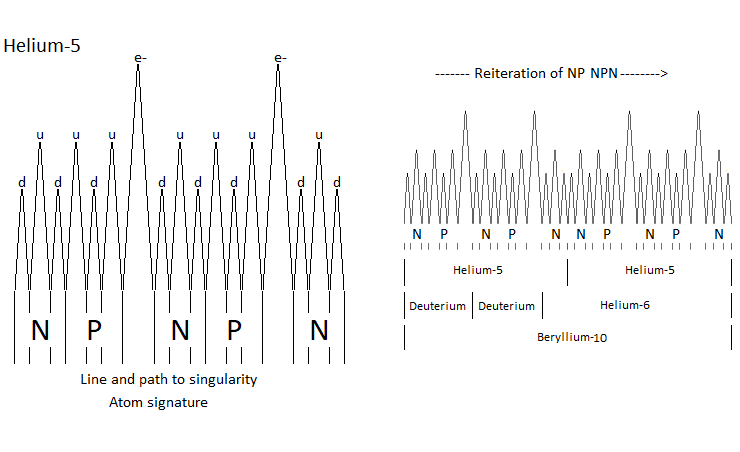The line spacing corresponds to the atomic signature as a function of the energy delivered by the particle. The smaller the energy in electron volts, the larger the line spacing. The absence of lines indicates that there are no particles in the field to be studied. Each line represents the path to the singularity by neutrinos as it descends, and responsible for the electrical charges generated as it ascends.

VI - Example structure & conclusion

The constitution of the physical laws as well as the result of this chaos of the universe until us suggests that it was premeditated.

The name of the main theory described in this paper is called Kartazion. Kartazion model of quantum physics and cosmological according to Arnaud Andrieu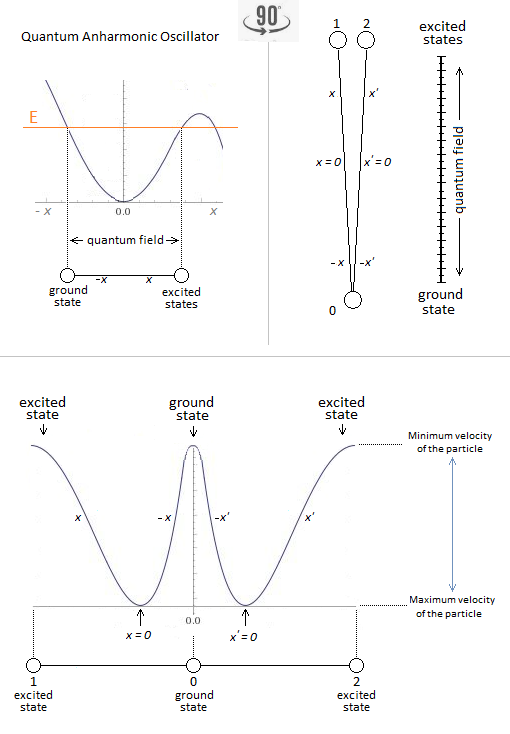End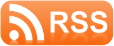• 11 Sep 2023-What Does Quantum Mechanics Say About Mind Powers? - Psychology Today
• 03 Jun 2023-Quantum dynamics of a driven parametric oscillator in a Kerr ... - Nature.com
• 21 Sep 2023-Astrophysics with continuous gravitational waves - Nature.com
• 23 Aug 2023-Quantum physics reveals the unity of the universe | Heinrich Päs - IAI
• 27 Aug 2023-CERN's Large Hadron Collider Makes Its First Observations of ... - science.slashdot.org
• 26 Jul 2023-Does Nothingness Exist? - Quanta Magazine
• 29 Jun 2023-A New Map of the Universe, Painted With Cosmic Neutrinos - Quanta Magazine
• 14 Jun 2023-Astronomers discover new link between dark matter and clumpiness ... - Science Daily
• 25 May 2022-Relativistic motions of spin-zero quantum oscillator field in a global ... - Nature.com
• 30 Jan 2023-Why More Physicists Are Starting to Think Space and Time Are ... - The Daily Beast

## Construction of the Universe with a Harmonic Oscillator

• Truncated mass divergence in a Mott metal | Proceedings of the ... - pnas.org *
• A quadratic time-dependent quantum harmonic oscillator | Scientific ... - Nature.com *
• Researchers detail how disorder alters quantum spin liquids, forming a new phase of matter - Phys.org *
• Bidirectional field-steering and atomic steering induced by a ... - Nature.com *
• A physics-based Ising solver based on standard CMOS technology - Phys.org *
• Connection Machine Joins All to All to Optimize Better - IEEE Spectrum *
• Dispersive readout of a silicon quantum device using an atomic ... - American Institute of Physics *
• Harnessing the Void: MIT Controls Quantum Randomness For the ... - SciTechDaily *
• Scientists make a quantum harmonic oscillator at room temperature - Phys.org *
• Quantum oscillator tech takes giant leap - Newswise *
• Quantum dynamics of a driven parametric oscillator in a Kerr ... - Nature.com *
• Hybrid quantum systems with high-T \$\$_c\$\$ superconducting ... - Nature.com *
• Postdoctoral Researcher in Physics job with UNIVERSITY OF ... - Times Higher Education *
• The symmetry in the model of two coupled Kerr oscillators leads to ... - Nature.com *
• Fractional quantum oscillator and disorder in the vibrational spectra ... - Nature.com *

## Universal Theory of Physical Science

• New Horizon Prize in Physics awarded to scientists chasing ... - Livescience.com *
• Opinion | Is There a Crisis in Cosmology? - The New York Times *
• Breakthrough Prize – Breakthrough Prize Announces 2024 ... - Breakthrough Prize *
• Time doesn't belong to physics | Jimena Canales - IAI *
• The Physics of Crowds - Nautilus - Nautilus Magazine *
• Ask Ethan: How can I grow up to study parallel Universes? - Big Think *
• Universe slows cosmic growth defying the theory of relativity - Interesting Engineering *
• Affine transformations accelerate the training of physics-informed ... - Nature.com *
• Physics - The Mystery of “Strange” Metals Explained - Physics *
• Dark Photons: The Key to Unraveling the Dark Matter Mystery? - SciTechDaily *

__________________________________

Be aware of fake accounts
using the Kartazion name.

See here

__________________________________